# Resources for Teaching  Complex Variables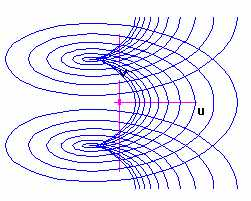Riemann Surface for the Logarithm Function.
Created using F(z) for Windows

This web site contains resources for individuals teaching an introductory, undergraduate course in complex variables. Over the years I've tried to create a series of activities, F(z) files, and Maple worksheets that can be used to create an active classroom learning atmosphere that replicates what I do in my calculus classes and that gives meaning to the various concepts from complex analysis.

## Site Contents:

[Activities] [ F(z) Programs]

## Activities

You'll need the free Adobe Acrobat Reader to view most of these activities.
• Euler's Identity, the Complex Exponential, and the Polar Form, Revisited: This is a brief activity in which students derive Euler's identity using Taylor series. They then plot a partial sum of the resulting series for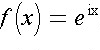as a vector using the tip to tail method of vector addition. A "spraling in" of the vectors illustrates the convergence of the series. Adapted from Visual Complex Analysis.
•  Mapping Properties of Complex-valued Functions In this activity students use F(z) and work in small groups to investigate mapping properties of various functions. Each group is given a particular function and a particular set of domains and is asked a series of questions that focus on mapping properties and that seek to compare and contrast properties of the function with its real counterpart. Each group then presents its findings to the rest of the class in the computer lab.
• The Amplitwist Principle This is a term I believe is due to Tristan Needham. The idea of this activity is for students to discover the idea that a complex-valued function is differentiable at a point provided it has a well-defined "amplitwist" there. An amplitwist is a composition of an amplification and a "twisting" or rotation. Under this identification, the derivative may be thought of locally as a linear transformation, and the tangent line approximation from calculus becomes an affine transformation. An F(z) file ( AMPLITW.FZW ) accompanies this activity.
• The Polya Vector Field is a useful tool for giving meaning to the basic concepts of complex varibles and has been written about extensively by Bart Braden. The idea is that one may associate to the complex-valued function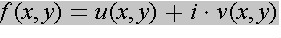the vector field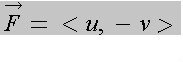. A plot of this vector field allows for visualization of a variety of ideas.
• Two student papers from 1998 that describe some of the properties of the Polya Field.:
• Brief activity on matching a Polya Field to its function formula. Polya Field Example
• Maple activity on differentiability and the Polya Field. If a function is analytic in some region, the divergence and curl of its associated Polya Field is identically zero. Hence, the Polya field has no sources and sinks and is irrotational.  Maple file (save by holding down shift/leftmouse simultaneously)  HTML version of Maple file
• Interpreting the Contour Integral This is an activity on the contour integral and the Polya Field. The real and imaginary parts of a contour integral measure the "flow" and "flux", respectively, of the Polya field along the contour.
• Maple activity on using the Polya Field and animation to visualize Taylor series convergence. I have students use this file in order to determine whether the Taylor series for a particular function converges at various points within a certain region. The objective is for students to discover on their own that the radius of convergence of a Taylor series is the distance from the center of the series to the nearest singularity of the function. Maple file (save by holding down shift/leftmouse simultaneously)  HTML version of Maple file (Note that the HTML version does not display the animation.)
• An Overview of the Contour Integral Class handout summarizing some properties of the contour integral.
• Cauchy's Theorem and Curve Deformation: In this activity students calculate the contour integral along a circle of radius two centered at the origin for a function having two poles inside this circle. At this point the students have not seen the Cauchy integral formula; instead they perform a partial fraction decomposition and use the fact that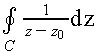, where C is a circle, is zero if z0 is outside this circle and is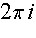if z0 is inside the circle. The circle is then moved so that it contains one singularity and then again so that it contains no singularities, and new values of the integral are quickly recalculated. An F(z) animation (using  DEFORM.FZW ) illustrates that as the circle moves in general, the contour integral can only take on three different values.
• ## Sample F(z) for Windows files:

For each F(z) file you wish to obtain, click on the file name. You should see a text file in your browser. Save this text file to disk, making sure to include the ".fzw" suffix at the end of the name you choose. Then open the file from within the F(z) program.
• COSINE.FZW: Illustrates the image under the cosine map of horizontal lines, vertical lines, and rectangular regions. Based upon an idea from Needham's book above.
• POLYAFLD.FZW: Draws the Polya Vector Field for the function f(z)=z^2. The Polya Field has been discussed extensively by Braden and allows for visualization of such ideas as differentiability (related to the divergence and curl of the field), the value of contour integrals (related to flux and flow along the contour), and the argument principle.
• DERIVDEF.FZW: Investigates differentiability near the origin for the function f(z)=z^2+z^5/norm(z^4). Different paths of approach are animated to show that (f(z)-f(0))/z has no limit as z approaches zero.
• AMPLITW.FZW: File that accompanies the activity The Amplitwist Principle. The "amplitwist" principle is the complex analog of the calculus idea of local linearity or linearization. If a function is differentiable at a point, then locally it behaves as an affine transformation of the plane.
• AVGVAL.FZW: Uses Gluchoff's interpretation of the contour integral as a vector average. The integral of a function f along an arc gamma is the length of the gamma multiplied by the average along gamma of f times the unit tangent vector.
• DEFORM.FZW: File that accompanies the activity Cauchy's Theorem and Curve Deformation. Animates the value of a contour integral as the location of the contour changes. The contour is a simple circle with changing center, and the integrand is a simple rational function with two poles.
• C_INTFRM.FZW: Allows for numerical approximation of an integral having integrand of the form f(z)/(z-z0). Using the mouse to estimate the value of the integral, together with a graphing calculator to estimate f(z0), students can discover the Cauchy Integral Formula..
• DISCCONV.FZW: Allows for discovery of the disk of convergence for the Taylor series of f(z)=1/(z^2+2) centered about the origin. The 10th and 20th partial sums of the Taylor series are plotted as vector fields. Inside the disk of radius sqrt(2) centered at the origin, the vector fields appear similar. Outside this same disk they appear quite different.
• UNIFCONV.FZW: Plots the error involved in using the partial sum of the Taylor series about z=0 for the function f(z)=1/(1-z). The errors are plotted using domains of concentric circles of increasing radii. As the radii of the circles approach 1, the error grows in magnitude, illustrating how convergence of the Taylor series is slowest near the boundary of the disk of convergence. I ask the students to do what they can to reduce the error. Most will simply increase the number of terms used in the partial sum, which of course doesn't really help. Usually a few students recognize the need to reduce the outer radius to a number slightly less than 1. This activity serves as a nice lead in to the idea of uniform convergence.
• REALINT.FZW: Investigates the integral of f(x)=cos(x)/(1+x^2) along the real line. The value of the integral f along a simple closed contour consisting of a segment along the real axis and a semicircle in the upper half plane are each independently illustrated. As the size of the contour increases, animation illustrates how the contribution to the value of the integral coming from the semicircle goes to zero.
• RIEMSURF.FZW: Uses the 4-D graphing features of F(z) to create the Riemann surface for the logarithm.
Click here for a detailed booklet in PDF format that gives instructions on how some of these F(z) files were created.

## Bibliography

• Beardon, Alan Iteration of Rational Functions , Springer-Verlag.
• Braden, Bart, "The Vector-Field Approach in Complex Analysis," from Visualization in Teaching and Learning Mathematics , MAA Notes #19, 1990, pp. 191-196.
• Braden, Bart, "Polya's Geometric Picture of Complex Contour Integrals," Mathematics Magazine , Volume 60, 1987, pp. 321-327.
• Braden, Bart, "Picturing Functions of a Complex Variable," College Mathematics Journal , Volume 16, 1985, pp. 63-72.
• Devaney, Robert, An Introduction to Chaotic Dynamical Systems , Addison-Wesley.
• Gluchoff, Alan, "Complex Power Series-A Vector Field Visualization," Mathematics Magazine , Volume 66, 1993, pp. 189-191.
• Gluchoff, Alan, "A Simple Interpretation of the Complex Contour Integral, The American Mathematical Monthly , Volume 98, 1991, pp. 641-644.
• Matthews, John and Howell, Russell, Complex Analysis for Mathematics and Engineering , Jones and Bartlett.
• Needham, Tristan, Visual Complex Analysis , Oxford University Press.
• Needham, Tristan, "The Geometry of Harmonic Functions," Mathematics Magazine , Volume 67, 1994, pp. 93-108.
• Newton, Tyre and Lafaro, Thomas, "On Using Flows to Visualize Functions of a Complex Variable," Mathematics Magazine , Volume 69, 1996, pp. 28-33.
• Polya, George and Latta, Gordon, Complex Variables , Wiley, 1974.